# Classification of orbit types using Neural Designer

The central goal is to design a model that makes useful classifications for the different orbit types.### Contents:

This example is solved with Neural Designer. To follow it step by step, you can use the free trial.

## 1. Application type

This is a classification project, since the variable to be predicted is categorical: AMO, APO, ATE. These orbit types are asteroid orbits, so the model will classify orbits according to the above asteroid orbit classes.

## 2. Data set

The first step is to prepare the data set, which is the source of information for the classification problem. For that, we need to configure the following concepts:

• Data source.
• Variables.
• Instances.

The data source is the file orbit_class.csv. It contains the data for this example in comma-separated values (CSV) format. The number of columns is 12, and the number of rows is 1722.

• a: Semi-major axis of the orbit in AU.
• e: Eccentricity of the orbit.
• i: Inclination of the orbit with respect to the ecliptic plane and the equinox of J2000 (J2000-Ecliptic) in degrees.
• w: Argument of perihelion (J2000-Ecliptic) in degrees.
• Node: Longitude of the ascending node (J2000-Ecliptic) in degrees.
• M: Mean anomaly at epoch in degrees.
• q: Perihelion distance of the orbit in AU.
• Q: Aphelion distance of the orbit in AU.
• P: Orbital period in Julian years.
• H: Absolute V-magnitude.
• MOID: Minimum orbit intersection distance (the minimum distance between the osculating orbits of the NEO and the Earth).
• class: AMO, APO, ATE used as the target.

The above target variables are asteroid orbits.

AMO is referring to Amor asteroids: near-Earth asteroids. The orbital perihelion of these objects is close to, but greater than, the orbital aphelion of Earth (a > 1.0 AU and 1.017 AU < q < 1.3 AU).

APO is referring to Apollo asteroids: near-Earth asteroid orbits which cross the Earth's orbit (a > 1.0 AU and q < 1.017 AU).

ATE refers to Aten asteroids: a dynamical group of asteroids whose orbits bring them into proximity with Earth. By definition, Atens are Earth-crossing asteroids (a<1.0 AU and Q>0.983 AU).

These differents asteroid orbits are shown in the following image: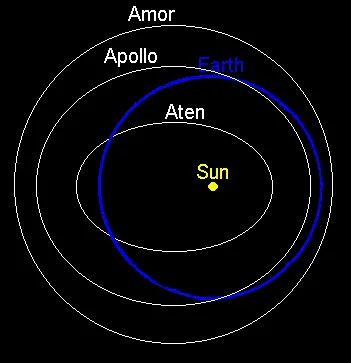Note that neural networks work with numbers. In this regard, the categorical variable "class" is transformed into three numerical variables as follows:

• AMO: 1 0 0.
• APO: 0 1 0.
• ATE: 0 0 1.

The instances are divided into training, selection, and testing subsets. They represent 60% (1034), 20% (344), and 20% (344) of the original instances, respectively, and are split at random.

We can calculate the distributions of all variables. The next figure is the pie chart for the orbit types.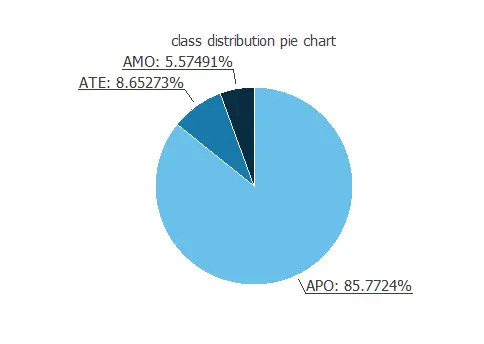As we can see, most of the samples are APO orbits.

Finally, the inputs-targets correlations might indicate to us what factors most influence.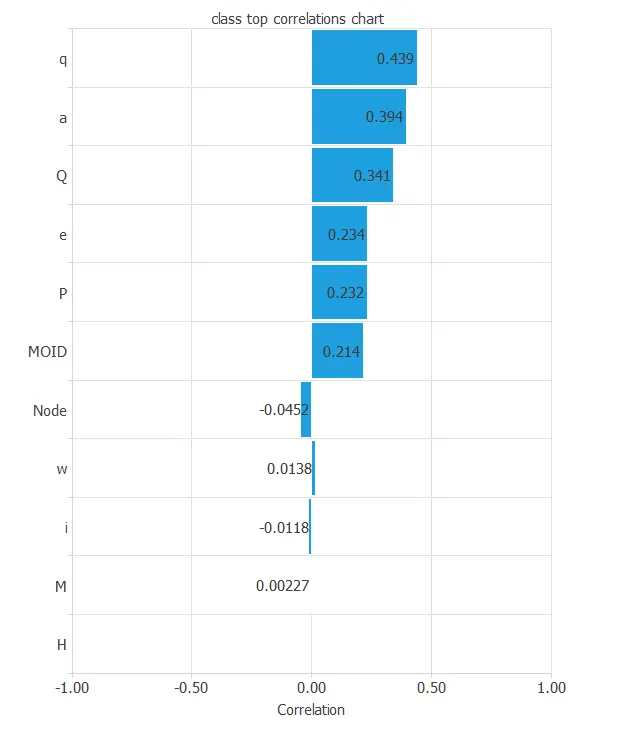Here, the most correlated variables with the classification are a, q, and Q, the semi-major axis, perihelion distance, and aphelion distance of the orbit, respectively. Also are not many correlated variables like M, mean anomaly, or H, absolute V-magnitude.

## 3. Neural network

The second step is to choose a neural network. For classification problems, it is usually composed by:

• A scaling layer.
• Two perceptron layers.
• A probabilistic layer.

The scaling layer contains the statistics on the inputs calculated from the data file and the method for scaling the input variables. Here the minimum and maximum method has been set. Nevertheless, the mean and standard deviation method would produce very similar results.

The number of perceptron layers is 1. This perceptron layer has 11 inputs and 3 neurons.

The probabilistic layer allows the outputs to be interpreted as probabilities. All outputs are between 0 and 1, and their sum is 1. The softmax probabilistic method is used here.

The neural network has three outputs since the target variable contains 3 classes (AMO, APO, ATE).

The next figure is a graphical representation of this classification neural network: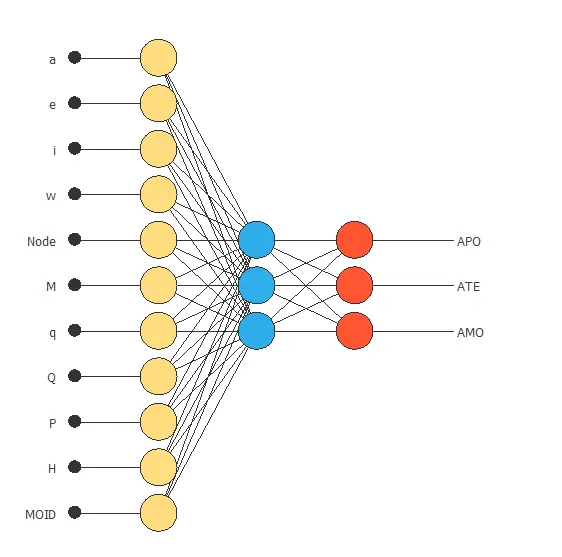Here, the yellow circles represent scaling neurons, the blue circles represent perceptron neurons, and the red circles represent probabilistic neurons. The number of inputs is 11, and the number of outputs is 3.

## 4. Training strategy

The fourth step is to set the training strategy, which is composed of:

• Loss index.
• Optimization algorithm.

The loss index chosen for this application is the normalized squared error with L2 regularization.

The error term fits the neural network to the training instances of the data set. The regularization term makes the model more stable and improves generalization.

The optimization algorithm searches for the neural network parameters which minimize the loss index. The quasi-Newton method is chosen here.

The following chart shows how the training and selection errors decrease with the epochs during the training process.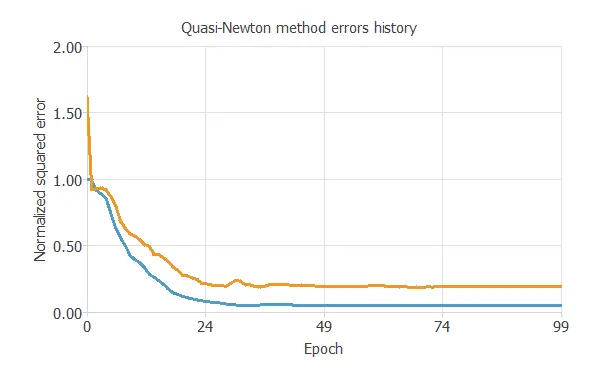The final values are training error = 0.0469 NSE (blue), and selection error = 0.189 NSE (orange).

## 5. Model selection

The objective of model selection is to find the network architecture with the best generalization properties, that is, that which minimizes the error on the selected instances of the data set.

Order selection algorithms train several network architectures with a different number of neurons and select that with the smallest selection error.

The incremental order method starts with a small number of neurons and increases the complexity at each iteration.

## 6. Testing analysis

The purpose of the testing analysis is to validate the generalization performance of the model. Here we compare the neural network outputs to the corresponding targets in the testing instances of the data set.

In the confusion matrix, the rows represent the targets (or real values) and the columns the corresponding outputs (or predictive values). The diagonal cells show the correctly classified cases, and the off-diagonal cells show the misclassified cases.

Predicted APO Predicted ATE Predicted AMO
Real APO 283 (84.0%) 0 (0.0%) 1 (0.3%)
Real ATE 1 (0.3%) 30 (8.7%) 0 (0.0%)
Real AMO 7 (2.0%) 0 (0.0%) 16 (4.7%)

As we can see, the number of instances that the model can correctly predict is 335 (97.4%), while it misclassifies is 9 (2.6%). This shows that our predictive model has a great classification accuracy.

## 7. Model deployment

The neural network is now ready to predict outputs for inputs that it has never seen. This process is called model deployment.

To classify a given orbit, we calculate the neural network outputs from the differents variables. For instance:

• a: 1.75 AU.
• e: 0.53.
• i: 13.35 degrees.
• w: 180.46 degrees.
• Node: 172.25 degrees.
• M: 180.73 degrees.
• q: 0.76 AU.
• Q: 2.75 AU.
• P: 2.44 yr.
• H: 19.94 .
• MOID: 0.02 AU.
• Probability of APO: 99.9%.
• Probability of ATE: ~0.0%.
• Probability of AMO: ~0.0%.

The neural network would classify the orbit as Apollo asteroid orbit for this particular case since it has the highest probability.

The mathematical expression of the trained neural network is listed below.

scaled_a = a*(1+1)/(17.81870079-(0.6369649768))-0.6369649768*(1+1)/(17.81870079-0.6369649768)-1;
scaled_e = e*(1+1)/(0.9560419917-(0.02542470023))-0.02542470023*(1+1)/(0.9560419917-0.02542470023)-1;
scaled_i = i*(1+1)/(75.41239929-(0.1460839957))-0.1460839957*(1+1)/(75.41239929-0.1460839957)-1;
scaled_w = w*(1+1)/(359.6629944-(0.5218380094))-0.5218380094*(1+1)/(359.6629944-0.5218380094)-1;
scaled_Node = Node*(1+1)/(359.855011-(0.1360419989))-0.1360419989*(1+1)/(359.855011-0.1360419989)-1;
scaled_M = M*(1+1)/(359.8250122-(0.05216519907))-0.05216519907*(1+1)/(359.8250122-0.05216519907)-1;
scaled_q = q*(1+1)/(1.060099959-(0.09279999882))-0.09279999882*(1+1)/(1.060099959-0.09279999882)-1;
scaled_Q = Q*(1+1)/(34.68000031-(0.9900000095))-0.9900000095*(1+1)/(34.68000031-0.9900000095)-1;
scaled_P = P*(1+1)/(75.22000122-(0.5099999905))-0.5099999905*(1+1)/(75.22000122-0.5099999905)-1;
scaled_H = H*(1+1)/(22-(14.10000038))-14.10000038*(1+1)/(22-14.10000038)-1;
scaled_MOID = MOID*(1+1)/(0.04998699948-(9.999999747e-06))-9.999999747e-06*(1+1)/(0.04998699948-9.999999747e-06)-1;

perceptron_layer_0_output_0 = tanh[ 0.998564 + (scaled_a*0.483063)+ (scaled_e*4.23015)+ (scaled_i*0.033193)+ (scaled_w*0.00368636)+ (scaled_Node*-0.0750109)+ (scaled_M*0.0156837)+ (scaled_q*4.51185)+ (scaled_Q*0.387602)+ (scaled_P*-0.48082)+ (scaled_H*-0.124104)+ (scaled_MOID*0.0301184) ];
perceptron_layer_0_output_1 = tanh[ 0.378967 + (scaled_a*0.0679598)+ (scaled_e*2.29236)+ (scaled_i*-0.259866)+ (scaled_w*-0.415081)+ (scaled_Node*-0.00389391)+ (scaled_M*-0.0223087)+ (scaled_q*-1.3599)+ (scaled_Q*0.12361)+ (scaled_P*-0.168758)+ (scaled_H*0.596372)+ (scaled_MOID*-0.376615) ];
perceptron_layer_0_output_2 = tanh[ -2.16292 + (scaled_a*1.04587)+ (scaled_e*0.149125)+ (scaled_i*0.107164)+ (scaled_w*-0.0590388)+ (scaled_Node*0.0606977)+ (scaled_M*-0.0259366)+ (scaled_q*5.8545)+ (scaled_Q*0.931787)+ (scaled_P*1.49335)+ (scaled_H*0.115587)+ (scaled_MOID*0.0850156) ];

probabilistic_layer_combinations_0 = -0.922149 +3.8602*perceptron_layer_0_output_0 +1.09178*perceptron_layer_0_output_1 -3.9471*perceptron_layer_0_output_2
probabilistic_layer_combinations_1 = 1.00764 -4.69034*perceptron_layer_0_output_0 +1.0006*perceptron_layer_0_output_1 -1.231*perceptron_layer_0_output_2
probabilistic_layer_combinations_2 = -0.0237251 +0.837826*perceptron_layer_0_output_0 -2.09368*perceptron_layer_0_output_1 +5.1967*perceptron_layer_0_output_2

sum_ = exp(probabilistic_layer_combinations_0 + exp(probabilistic_layer_combinations_1 + exp(probabilistic_layer_combinations_2;

APO = exp(probabilistic_layer_combinations_0)/sum_;
ATE = exp(probabilistic_layer_combinations_1)/sum_;
AMO = exp(probabilistic_layer_combinations_2)/sum_;


We have just built a predictive model to determine the possible asteroid orbit type.